Thank you for visiting nature.com. You are using a browser version with limited support for CSS. To obtain the best experience, we recommend you use a more up to date browser (or turn off compatibility mode in Internet Explorer). In the meantime, to ensure continued support, we are displaying the site without styles and JavaScript.

Magnonic Band Engineering by Intrinsic and Extrinsic Mirror Symmetry Breaking in Antidot Spin-Wave Waveguides

Abstract

We theoretically study the spin-wave spectra in magnonic waveguides periodically patterned with nanoscale square antidots. We show that structural changes breaking the mirror symmetry of the waveguide can close the magnonic bandgap. The effect of these intrinsic symmetry breaking can be compensated by adjusted asymmetric external bias magnetic field, i.e., by an extrinsic factor. This allows for the recovery of the magnonic bandgaps. The described methods can be used for developing parallel models for recovering bandgaps closed due to a fabrication defect. The model developed here is particular to magnonics, an emerging field combining spin dynamics and spintronics. However, the underlying principle of this development is squarely based upon the translational and mirror symmetries, thus, we believe that this idea of correcting an intrinsic defect by extrinsic means, should be applicable to spin-waves in both exchange and dipolar interaction regimes, as well as to other waves in general.

Introduction

The possibilities for fabrication of metallic magnetic materials with nanoscale precision have opened the pathway for tailoring the dispersion of high-frequency spin-waves (SWs) and development of magnonics - a new innovative field of research covering various aspects of magnetization dynamics1,2,3. This also leads to the emergence of magnonic crystals (MCs), which are magnetic counterparts of photonic and phononic crystals4,5,6 and constitute the fundamental building blocks of magnonics. Recently the first bi-component MCs have been realized at the nanoscale and the opening of magnonic gaps was proven experimentally7,8. Two-dimensional antidots lattices formed by periodic array of holes in ferromagnetic film can be much easily fabricated. These systems have been intensively studied in recent years on length scales ranging from micrometers down to few tens of nanometres9,10,11,12,13,14. For antidot lattices with large period, the inhomogeneity of the internal magnetic field is decisive for the formation of the magnonic band structure15. With the decreasing period of antidot lattice the Brillouin zone (BZ) border will move to larger wave-vectors and the exchange interactions at some point will start to play a primary role in the formation of magnonic band structure.

Prototypes of basic magnonic devices have already been demonstrated to be promising for technological applications1,16,17,18,19 but the scaling down of magnonic elements to tens of nanometres in size and tens to hundreds of GHz of operating frequencies20,21,22,23 are still a challenge. Waveguides for SWs, which are deemed to be of vital importance in most magnonic devices1, have been realized experimentally in various ways; such as, in the form of flat stripes and having filtering properties due to periodically modulated width or based on missing-row defect in two-dimensional thin-film MCs2,11,24,25,26,27,28,29. But so far, experiments are only done for SWs in the frequency range up to few GHz. However, with the recent advances in fabrication techniques, it has become feasible to pattern with resolution below 10 nm. For example, the spot size during focused ion beam lithography can go below 10 nm with low (≈30 pA) ion current14,30,31,32,33. To predict properties of magnonic devices at nanometer length scale, more basic research needs to be conducted. Therefore, theoretical investigation of the SW waveguides operating in the range of tens to hundreds of GHz is a frontier field of research.

Recently, micromagnetic simulations (MSs) were used to show that periodic waveguides have filter properties due to the opening of magnonic gaps in the SW spectrum at high frequencies34,35,36. In this paper we investigate the influence of the fundamental property of symmetry on the magnonic band structure. We study how loss of mirror symmetry within an one-dimensional nanoscale antidot lattice waveguide (ADLW) may affect the magnonic bandgap. When this symmetry exists then based on their profiles with respect to the central longitudinal axis, the SW spectra can be separated into two groups: symmetric modes and anti-symmetric modes. The breaking of the mirror symmetry will automatically make the classification impossible. We will study two types of the symmetry breaking mechanisms: categorizing them as intrinsic and extrinsic. To demonstrate the generality of the methods discussed here, we have considered two intrinsic factors and two different kinds of field profiles: stepped or ramped (extrinsic factors). The intrinsic factors discussed here are the shape of the antidots and their positions within the ADLW. The question is: how do these changes influence the magnonic spectra and the existing bandgaps? Also, how “big” the symmetry breaking needs to be in order to close the gaps? The answers to these questions are very important for the applications of nanoscale SW waveguides in magnonic signal processing and also from the point of view of the basic research, as it concerns fundamental properties of a diverse group of systems. We address these questions in this article and go even further in terms of magnonic band engineering. We will study the possibility of compensating the changes introduced by the structural modifications in the magnonic spectra by modifying the bias magnetic field. We will demonstrate how collapsed bandgaps in asymmetric waveguides can be restored by the application of the asymmetric bias magnetic field. The extent of this restoration is also studied.

Results

Magnonic band structure in symmetric and asymmetric ADLW

We study the symmetric and asymmetric magnonic waveguides based on the antidot lattice structure shown in Fig. 1. It has the form of a thin (thickness u = 3 nm) and infinitely long permalloy (Ni80Fe20) stripe with a single row of square holes (s = 6 nm antidots) disposed periodically along the central line. The stripe width and the lattice constant are fixed at 2 × w + s = 45 nm and a = 15 nm, respectively. The row of holes is placed at the distance w = 19.5 nm from the top (and bottom) edge of the stripe in the case of the symmetric ADLW. A bias magnetic field, strong enough to saturate the sample (μ0H0 = 1 T), is applied along the length of the stripe. The saturation magnetization Ms = 0.8 × 106 A/m, the exchange length λex = 5.69 nm and the gyromagnetic ratio γ = 175.9 GHz/T were assumed in calculations.

We start our investigation with the symmetric ADLW (Fig. 1)38. The dispersion relations of SWs in the symmetric ADLW is shown in Fig. 2. The results of the OOMMF simulations are shown in Fig. 2 (a) and of the PWM in Fig. 2c (black solid lines). The agreement between results from these two methods is satisfactory. The presence of two magnonic bandgaps (of about 4 GHz each) is evident and they are marked in yellow. The origins of these two bandgaps were found to be different. The first one opens at the BZ boundary due to the Bragg reflection of SWs, while the second gap opens up within the BZ38. It was shown that this splitting of the bands within the BZ is due to the anti-crossing between two families of modes34, those with and without a nodal line in the upper and lower parts of the ADLW (see the first row of profiles in the bottom panel of Fig. 2). We showed in Ref. 38 that the pinning at the edges of Py (at the waveguide edges and at edges of antidots) is crucial for the existence of these magnonic gaps.

The structure investigated above has a mirror symmetry with respect to the central axis of the ADLW. Thus, the 1st and the 3rd modes are symmetric while the 2nd and the 4th are antisymmetric. The frequencies of first two modes (symmetric and antisymmetric one) are degenerate in the entire wavevector regime and their maps of square of the amplitude of these modes are identical (see the first and third rows of profiles for BZ center and border in the bottom panel of Fig. 2). The degeneracy of symmetric and antisymmetric oscillations in the waveguide points at very weak coupling of oscillations localized in the upper and the lower parts of the ADLW (in the two equivalent sub-waveguides, Fig. 1). The shift of the row of antidots from the central line will break the mirror symmetry of the ADLW. If this shift is in +y direction, the upper and lower sub-waveguides will become narrower and wider, respectively. Frequencies of modes localized in the two sub-waveguides will split, with one mode shifted up and the other shifted down on the frequency scale. The dispersion relations of SWs in asymmetric ADLWs, obtained by shifting the row of antidots by Δw = 1 nm and 0.9 nm upward, calculated using OOMMF and PWM, are presented in Figs. 2b and c (red lines), respectively. (In OOMMF slightly larger value of Δw were used because of the limitations of the discretization mesh and time needed for simulations.)

We see that a shift of the antidots row (along the width of ADLW) by only 2% of 2w + s is enough to close both magnonic gaps. At the BZ center, the first (second) mode center has an amplitude concentrated in the wider (narrower) part of the ADLW of width w + Δw (w − Δw) (see profiles in the second row in Fig. 2 at the bottom). The modes 3′ and 4′ at the BZ boundary originate from modes 1′ and 2′, respectively due to the folding from the neighboring BZ. Therefore, their profiles of amplitudes are quite similar. Typically, lower frequency modes are concentrated in wider regions of space. It means that two lower (higher) modes must be concentrated in wider (narrower) ADLW. Note that, the oscillations of the magnetization amplitude for the modes at the BZ boundary are related to the shifting of the phase of the Bloch waves with the period of the lattice. When Δw = 0, modes 1′ and 2′ are concentrated in relatively larger regions (between the antidots) in the two sub-waveguides when compared to the coverage of modes 3′ and 4′ (directly above or below the antidots). However, when the mirror symmetry is lost (Δw≠0), 1′ and 3′ cover the larger regions between the antidots while 2′ and 4′ are limited to the smaller regions directly above or below the antidots in the two sub-waveguides. Further, 3′ and 4′ are on a narrower sub-waveguide as compared to 1′ and 2′. This makes the spatial distribution of 2′ and 3′ comparable in shape and expanse. Thus these two modes have similar frequencies at the BZ boundary which, in turn, leads to the collapse of the first magnonic bandgap. A very similar mechanism is responsible for the closing of the second gap as well; even though the origin of this gap is different and the respective changes of frequencies of the third and the fourth bands are larger. As we mentioned before, the second gap appears at the anti-crossing of the modes with different quantization across the width of ADLW. The modes 3′ and 4′ have no horizontal nodal line inside of each sub-waveguides whereas the modes 3 and 4 have one for each part of the ADLW.

The bandgap widths as a function of Δw are shown in Fig. 3 (a). The widths decrease monotonously with increasing Δw. The slope for the second gap is larger leading to its complete collapse at Δw = 0.45 nm, while the first gap exists up to 0.8 nm. We note that the shift of the antidots row does not change the translational periodicity in the structure. Thus the observation of magnonic bandgap closing shown in Fig. 3 (a) is purely related to the loss of the mirror symmetry of ADLW and associated movement of different modes.

We now demonstrate that breaking the mirror symmetry by extrinsic means can also lead to splitting of the bands and closing of magnonic gaps. In Fig. 4 we show PWM results (dashed green lines) with the additional magnetic field ΔH00ΔH0 = 180 mT) applied (a) parallel and (b) antiparallel to the original bias H0, in the upper part of the symmetric ADLW. The black solid lines mark the magnonic band structure for the homogeneous magnetic field, i.e., the same as in Fig. 2 c). The parts of the ADLW where the increased or reduced bias magnetic fields were applied were 18 nm wide from the closest ADLW edge. Similar results were also obtained from simulations (not shown). The parts of the ADLW with changed (increased or decreased) bias magnetic field are marked with green color in the insets of Fig. 4. From Fig. 4, we can see that the (a) increase or (b) decrease of the bias magnetic field splits frequency bands by shifting the position of some modes up or down, while other frequency modes remain unchanged. The squared amplitudes of the SWs pertaining to the first two modes (1 and 2) are calculated at the BZ centre and are shown at the left and right of Fig. 4. A selective population distribution, predicated upon the changed external field, is clearly evident amongst these modes. The increase (decrease) of the bias magnetic field in the upper half of the ADLW increase (decrease) the frequency of the modes localized in this sub-waveguide. It is worth noting that an uniform change of the magnetic field will shift the whole spectra but preserve the bandgaps in the structure.

Compensation of the effect of an intrinsic symmetry breaking

We have just shown that the magnonic spectra, especially the magnonic bandgaps for the considered ADLW are sensitive towards loss of its (intrinsic or extrinsic) mirror symmetry. We now investigate if it is possible to compensate the effect of an intrinsic symmetry breaking in an ADLW by an extrinsic factor. In our case it will be a compensation of the effect of the structural asymmetry on the magnonic band structure (and magnonic bandgaps) by asymmetric bias magnetic field. The answer will begin from the development of an analytical model.

We showed that the amplitudes of modes from the first four magnonic bands in asymmetric ADLW concentrate mainly at the top or bottom part of the structure (see the square of the amplitude in Figs. 2 and 4). This allows for a qualitative explanation of the observed changes in magnonic band structure by a model of two sub-waveguides (in the upper and lower parts of the waveguide), which are weakly coupled through a row of antidots. This observation lets us also to make the estimation of a compensation of the symmetry breaking mechanisms. We will discus first the effect of the changes of the width and bias magnetic field in a single waveguide (WG) on the dispersion relation of SWs.

In the homogeneous WG the solutions of the linearized LLG equation (with damping neglected) can be written in the following form: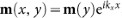, where kx is the wave-vector of the SW along the WG and m(y) describes the dependence of the amplitude of dynamical components of the magnetization m across the WG width (we assume the uniform magnetization across the WG thickness, which is much less than the width). The solutions can be estimated as: m(y) ≈ sin(κy), cos(κy) where the transversal component of the wave-vector κ = (n + 1)π/weff is quantized (n = 0, 1, 2, … counts the number of nodal lines across the WG width). For strong but not ideal pinning the effective width weff = wd/(d − 2) depends on the pinning parameter d, which determines the boundary conditions for magnetization and gives also a possibility to include the dipolar effects into the model40. It varies in general from 0 to ∞ for the transition from unpinned to pinned boundary conditions. The pinning parameterdepends both on the material and structural parameters (Ks denotes the surface anisotropy). It accounts for both the exchange and the dipolar interactions. For d ≈ ∞, as in our numerical calculations, n = 0 means no nodal line in the upper or lower part of the ADLW (see, Fig. 2; modes 1 and 2), n = 1 denotes a single nodal line (see, Fig. 2; modes 3 and 4), etc. The dispersion relation of SWs in the WG can be written in the form40,41: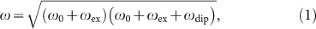where ω is the angular frequency of SWs. ω0 = γμ0H0,,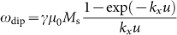denote the contributions from external, exchange and dipolar fields, respectively.

The estimations of changes in SW dispersion relation resulting from the changes of w or H0 can be done by calculation of the full differential of the function ω = ω(w, H0). It will allow one to derive the relation between small changes of ΔH0 and Δw, for which the desired compensation between intrinsic and extrinsic symmetry breaking is obtained, i.e., when dω(w, H0) = 0: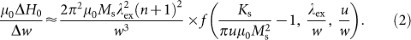This ratio, having units of T/m, describes how much extra asymmetric magnetic field needs to be added to compensate for the shift in the row of the antidots. The function: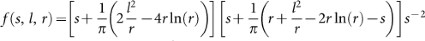depends on three dimensionless parameters: s–the relative strength of the surface anisotropy, l–ratio between exchange length and the width of the WG and r–the aspect ratio of the WG. The values of f(s, l, r) with big absolute value of Ks, refer to the regime of strong pinning. Note that Eq. (2) does not depend on kx, which means that it should be fulfilled for any wave-vector.

In our ADLW, we have two sub-waveguides separated by the antidots row. When we shift the row of the antidots by Δw along positive y direction, the width of the upper sub-waveguide decreases by Δw and the width of the lower sub-waveguide increases by the same amount. This causes the higher and lower frequency modes to become concentrated in the narrower and wider sub-waveguide, respectively. To compensate for these changes in the dispersion relation by a bias magnetic field we need to do the opposite. According to Eq. (2) we need to apply different bias magnetic fields to upper and lower sub-waveguides. The dependence of the magnonic gap width under asymmetric bias magnetic field of the step-like shape applied to the ADLW; i.e., in the upper part of the ADLW bias magnetic field is H0 + ΔH0, while in the lower part of ADLW is H0 − ΔH0, calculated with PWM is shown in Fig. 3 (b). We can see decrease in the gap widths with increasing ΔH0, similar to the changes observed with increasing Δw.

After these estimations we perform the PWM calculations. The results are presented in Fig. 5 (b) and (c) for ΔH0 to recover the first and the second magnonic gaps in the asymmetric ADLW (i.e., when Δw = 0.9 nm and 0.5 nm), respectively (see Fig. 3 (a)). It is interesting that we found it possible to recover the first and the second magnonic gaps but with different values of the ratio. The analytical values of this ratio (calculated from the Eq. (2) with w = 18.5 nm, i.e., the distance between pinned layers used in MS) for the ideal pinning (f(s, l, r) = 1) for the first gap (when n = 0) and the second one (n = 1) are 101 mT/nm and 406 mT/nm, respectively. To validate our predictions we performed MSs for μ0ΔH0 = 105 mT and Δw = 1 nm. The simulation results are shown in Fig. 5 (a) with the first frequency gap opened and in good agreement with the PWM calculations shown in Fig. 5 (b). Although, the second bandgap is formed due to the anti-crossing of the n = 0 and n = 1 modes, at Δw = 0.5 nm the splitting of the n = 1 dominates (see Figs. 2 (b) and (c)). Hence, in order to open the second gap we have to target the shifts for the bands with a single nodal line (n = 1) by applying the field for whichis about 4 times bigger than that for the first gap (410 mT/nm). This confirms the applicability of Eq. (2) with a square dependence on n + 1. The profiles of SWs (compare bottom panels of Fig. 2 and Fig. 5) further establish the restoration of amplitude distribution by extrinsic compensation. The presented results proved that the asymmetric bias magnetic field can reduce the effect of the intrinsic symmetry breaking introduced by the shifting of the position of antidots on magnonic spectra. Small differences in the extent of bandgap recovery obtained from numerical calculations and the analytical model, show that the pinning in the middle of ADLW is not perfect.

Our predictions should also be applicable to ADLWs where the loss of the mirror symmetry has occurred due to a change in a different intrinsic parameter. In order to establish the same, we now perform calculations for ADLW with rectangular antidots. The new ADLW structure is shown at the top row of Fig. 6. The ADLW consists of the rectangular antidot row with dimensions 6 nm × 4.5 nm, along the waveguide and across its width, respectively. The sub-waveguides formed on both sides of the antidots have now different width of w = 19.5 and w + Δw = 21 nm. Study of this kind of asymmetry can be of practical importance, because such unintended defects can occur during the design or fabrication stages. The magnonic band structures calculated with PWM and OOMMF for this ADLW are shown in the Fig. 6 (a). We can see that the mirror symmetry breaking by the decrease of antidots size across the waveguide width results in the splitting of magnonic bands and consequently the collapse of bandgaps in a manner similar to the results presented in Fig. 2 (b). The analytical formula Eq. (2) still can be used to estimate the bias magnetic field necessary to reopen magnonic bandgap in the spectra. According to Fig. 6 (a) we need to increase the frequency of the first and third modes without affecting the second and forth modes. According to our models, we should be able to achieve this simply by increasing the magnetic field in the wider (21 nm wide) part of the ADLW by μ0ΔH0 = 0.02 T. The result of the calculations for the step-like magnetic field is shown in Fig. 6 (b). The first magnonic bandgap has almost the same width as for the symmetric waveguide. The results of the PWM calculations are confirmed by MSs, which are shown in Fig. 6 (a) and (b) as color maps. The good agreement is found.

Discussion

We have shown that a small mirror symmetry breaking in ADLW by the shift of the antidots row from the waveguide axis or by an asymmetric change of their shape (i.e., by changes, which leave the discrete translational symmetry of the lattice intact) can result in closing of magnonic bandgaps in the range of the spectra determined mainly by exchange interactions. We observed that the loss of symmetry causes a redistribution of the amplitude associated with different SW modes in the physical space of the ADLW. This results in the movement of modes in the SW spectrum. Although, the two bandgaps observed and discussed here have different origins, their collapse is demonstrably a direct result of the loss of the mirror symmetry and the associated redistribution of SW amplitude.

Moreover, we have shown that the magnonic bandgap in the asymmetric ADLW can be reopened by an asymmetric bias magnetic field of a step-like profile across the ADLW width. With the help of an analytical model we were able to extract the main parameters responsible for closing the gap and its reopening by the external magnetic field. We presented that two magnonic bandgaps of different origins can be selectively reopened in the asymmetric waveguides by this way. We have shown here with an analytical model and also in the previous papers (Refs. 38, 42 and 43), that the detailed shape of antidots and random defects do not play significant roles in effects studied in the manuscript. Our results can be crucial for practical realization of SW waveguides for magnonic applications in high frequencies, because precise mirror symmetry is difficult to achieve on such small scales, leading to deviations form the ideal structure. The intrinsic and extrinsic symmetry breaking or its compensation can also be exploited to tailor the magnonic band structure or manipulate active and inactive waveguide modes, which couple to the external fields45,46 in a similar way as was predicted for plasmonic metamaterials47.

The experimental proof of the compensation effect proposed here with the step-like profile of the bias magnetic field is challenging. More feasible for experimental realization will be a continuous change of the bias magnetic field. We propose to use a ramp-like profile of the magnetic field44: H = H0 + 2ΔH0(2y + Δw), where y = 0 corresponds to the ADLW center. The values of Δw and ΔH0 can take the same values as for the step-like profile of magnetic field considered above. The results of PWM calculations for the ΔH0 and Δw taken in the calculations presented above are shown in Fig. 5 in right insets. These results were also confirmed by MSs. We have found, that this kind of field acts similar to the field with step-like profile, when its value is normalized to the same average value as the step-like field for corresponding sub-waveguides (the aforementioned formula for ramp-like field meets this criteria).

The development of the analytical model presented here was made possible solely by dint of the fundamental properties of discrete translational and mirror symmetries of a crystal lattice. Thus, the main conclusions should not be limited to the particular cases investigated here and it should be possible to extend this idea to other SW waveguides, including those with larger dimensions, or to other types of waves. In the former case the inhomogeneous demagnetizing field, anisotropy of magnetostatic SW dispersion relation48 and multimode character of waveguides49 have to be taken into account. Thus, further investigation is necessary. The compensation effects proposed here should find applications also in other systems, like electrons propagating in a periodically patterned graphene nano-ribbon by the external electric field50,51,52, or in photonic, plasmonic and phononic waveguides although with tailored electric and elasticity fields, respectively.

Methods

The calculations of the magnonic band structure are performed with the finite difference method MS and the PWM, with OOMMF37 and a Fortran code developed by authors, respectively. Both methods solve the Landau-Lifshitz-Gilbert (LLG) equation: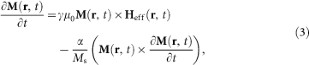where r and t are position vector and time, respectively. μ0 is the permeability of vacuum. The first term on the right hand side is related to the torque inducing precession of the magnetization M and the second one describe the damping process (α denotes the damping constant). The damping is neglected in PWM calculations and included in MS (α = 10−4). The effective magnetic field Heff here consists of the bias magnetic field H0, demagnetizing field and exchange field. The pinned dynamical components of the magnetization vector were assumed at Py/air interfaces in calculations with both methods. The pinning in OOMMF was introduced by fixing magnetization vector in all cells of the discretization mesh, which border the antidots, i.e., for the width 0.5 nm along y axis. (In MS the discrete mesh size of 1.5 × 0.5 × 3 nm along x, y and z axis, respectively, were used. The MS were performed for 4 ns. In the PWM we use 961 plane waves.) Further details on obtaining the SW dispersion relations by analyzing the results of MSs are discussed in Ref. 39. In the PWM the pinning is applied exactly at the edges of Py. Due to small thickness of the ADLW, uniform SW profile across the thickness is assumed. Both methods were already used in the calculations of the SW dynamics and proved to give correct results10,12,38,39.

References

1. Kruglyak, V. V., Demokritov, S. O. & Grundler, D. Magnonics. J. Phys. D: Appl. Phys. 43, 264001–14 (2010).

2. Lenk, B., Ulrichs, H., Garbs, F. & Münzenberg, M. The building blocks of magnonics. Phys Rep. 507, 107–136 (2011).

3. Magnonics from Fundamentals to Applications, Eds. Demokritov, S. O. & Slavin, A. N. (Topics in Applied Physics 125, Springer, 2013).

4. Nikitov, S. A., Tailhades, Ph. & Tsai, C. S. Spin waves in periodic magnetic structures-magnonic crystals. J. Magn. Magn. Mater. 236, 320–330 (2001).

5. Puszkarski, H. & Krawczyk, M. Magnonic crystals the magnetic counterpart of photonic crystals. Solid State Phenomena 94, 125–134 (2003).

6. The Physics of Phonons, Eds. Srivastava, G. P., Adam Hilger, 1990).

7. Wang, Z.-K. et al. Nanostructured magnonic crystals with size-tunable bandgaps. ACS Nano 4, 643–648 (2010).

8. Tacchi, S. et al. Forbidden bandgaps in the spin-wave spectrum of a two-dimensional bicomponent magnonic crystal. Phys. Rev. Lett. 109, 137202–5 (2012).

9. Pechan, M. J., Yu, C., Compton, R. L., Park, J. P. & Crowell, P. A. Direct measurement of spatially localized ferromagnetic-resonance modes in an antidot lattice. J. Appl. Phys. 97, 10J903–6 (2005).

10. Neusser, S. et al. Tunable metamaterial response of a Ni80Fe20 antidot lattice for spin waves. Phys. Rev. B 84, 184411–11 (2011).

11. Neusser, S. et al. Anisotropic propagation and damping of spin waves in a nanopatterned antidot lattice. Phys. Rev. Lett. 105, 067208–4 (2010).

12. Tacchi, S. et al. Mode conversion from quantized to propagating spin waves in a rhombic antidot lattice supporting spin wave nanochannels. Phys. Rev. B 86, 014417–12 (2012).

13. Hu, C.-L. et al. Field tunable localization of spin waves in antidot arrays. Appl. Phys. Lett. 98, 262508–3 (2011).

14. Mandal, R. et al. Optically induced tunable magnetization dynamics in nanoscale Co antidot lattices. ACS Nano 6, 3397–3403 (2012).

15. Zivieri, R. et al. Bragg diffraction of spin waves from a two-dimensional antidot lattice. Phys. Rev. B 85, 012403–6 (2012).

16. Serga, A. A., Chumak, A. V. & Hillebrands, B. YIG magnonics. J. Phys. D: Appl. Phys 43, 264002–16 (2010).

17. Macia, F., Kent, A. D. & Hoppensteadt, F. C. Spin-wave interference patterns created by spin-torque nano-oscillators for memory and computation. Nanotechnology 22, 095301 (2010).

18. Khitun, A. Multi-frequency magnonic logic circuits for parallel data processing. J. Appl. Phys. 111, 054307–9 (2012).

19. Chumak, A. V. et al. All-linear time reversal by a dynamic artificial crystal. Nature Communications 1, 141–5 (2010).

20. Bonetti, S., Muduli, P., Mancoff, F. & Akerman, J. Spin torque oscillator frequency versus magnetic field angle: The prospect of operation beyond 65 GHz. Appl. Phys. Lett. 94, 102507–3 (2009).

21. Prokopenko, O., Bankowski, E., Meitzler, T., Tiberkevich, V. & Slavin, A. Spin-torque nano-oscillator as a microwave signal source. IEEE Magnetic Letters 2, 3000104 (2011).

22. Madami, M. et al. Direct observation of a propagating spin wave induced by spin-transfer torque. Nature Nanotechnology 28, 635–638 (2011).

23. Satoh, T. et al. Directional control of spin-wave emission by spatially shaped light. Nature Photonics 6, 662–666 (2012).

24. Kozhanov, A. et al. Dispersion in magnetostatic CoTaZr spin waveguides. Appl. Phys. Lett. 94, 012505–3 (2009).

25. Demidov, V. E. et al. Excitation of short-wavelength spin waves in magnonic waveguides. Appl. Phys. Lett. 99, 082507–3 (2011).

26. Bai, L., Kohda, M. & Nitta, J. Observation of spin wave modes depending on a tunable periodic magnetic field. Appl. Phys. Lett. 98, 172508–3 (2011).

27. Clausen, P. et al. Mode conversion by symmetry breaking of propagating spin waves. Appl. Phys. Lett. 99, 162505–3 (2011).

28. Duerr, G., Thurner, K., Topp, J., Huber, R. & Grundler, D. Enhanced transmission through squeezed modes in a self-cladding magnonic waveguide. Phys. Rev. Lett. 108, 227202–5 (2012).

29. Schwarze, T. & Grundler, D. Magnonic crystal wave guide with large spin-wave propagation velocity in CoFeB. Appl. Phys. Lett. 102, 222412–4 (2013).

30. Rahman, F. H. M. et al. The Prospects of a Subnanometer Focused Neon Ion Beam. Scanning 34, 129–6 (2012).

31. Sidorkin, V. et al. Sub-10-nm nanolithography with a scanning helium beam. J. Vac. Sci. Technol. B 27, L18–3 (2009).

32. Siegfried, T. et al. Fabrication of sub-10 nm gap arrays over large areas for plasmonic sensors. Appl. Phys. Lett. 99, 263302–3 (2011).

33. Bourdillon, A. J., Williams, G. P., Vladimirsky, Y. & Boothroyd, C. B. Near-field x-ray lithography to 15 nm. Proc. SPIE 5374, 546 (2011).

34. Lee, K.-S., Han, D.-S. & Kim, S.-K. Physical origin and generic control of magnonic bandgaps of dipole-exchange spin waves in width-modulated nanostrip waveguides. Phys. Rev. Lett. 102, 127202–4 (2009).

35. Ma, F. S. et al. Micromagnetic study of spin wave propagation in bicomponent magnonic crystal waveguides. Appl. Phys. Lett. 98, 153107–3 (2011).

36. Kim, S.-K. Micromagnetic computer simulations of spin waves in nanometre-scale patterned magnetic elements. J. Phys. D: Appl. Phys. 43, 264004–26 (2010).

37. Donahue, M. & Porter, D. G. OOMMF Users guide, Version 1.0, Interagency Report NISTIR 6376 (NIST, Gaithersburg, 1999) at http://math.nist.gov/oommf/.

38. Kłos, J. W. et al. Effect of magnetization pinning on the spectrum of spin waves in magnonic antidot waveguides. Phys. Rev. B 86, 184433–8 (2012).

39. Kumar, D., Dmytriiev, O., Ponraj, S. & Barman, A. Numerical calculation of spin wave dispersions in magnetic nanostructures. J. Phys. D: Appl. Phys. 45, 015001–11 (2012).

40. Guslienko, K. Yu. & Slavin, A. N. Boundary conditions for magnetization in magnetic nanoelements. Phys. Rev. B 72, 014463–5 (2005).

41. Stancil, D. D. & Prabhakar, A. Spin waves (Springer, 2009).

42. Barman, A. Control of magnonic spectra in cobalt nanohole arrays: the effects of density, symmetry and defects. J. Phys. D: Appl. Phys. 43, 195002–7 (2010).

43. Kumar, D. et al. Effect of hole shape on spin-wave band structure in one-dimensional magnonic antidot waveguide. J. Appl. Phys. 114, 023910–6 (2013).

44. Cho, Z. H. & Yi, J. H. Planar surface gradient coil. Concept Magnetic Res. 7, 95–114 (1995).

45. Vlaminck, V. & Bailleul, M. Spin-wave transduction at the submicrometer scale: Experiment and modeling. Phys. Rev. B 81, 014425–13 (2010).

46. Au, Y., Dvornik, M., Dmytriiev, O. & Kruglyak, V. V. Nanoscale spin wave valve and phase shifter. Appl. Phys. Lett. 100, 172408–4 (2012).

47. Christ, A., Martin, O. J. V., Ekinci, Y., Gippius, N. A. & Tikhodeev, S. G. Symmetry breaking in a plasmonic metamaterial at optical wavelength. Nano Lett. 8, 2171–5 (2008).

48. Gieniusz, R. et al. Single antidot as a passive way to create caustic spin-wave beams in yttrium iron garnet films. Appl. Phys. Lett. 102, 102409–4 (2013).

49. Birt, D. R. et al. Diffraction of spin waves from a submicrometer-size defect in a microwaveguide. Appl. Phys. Lett. 95, 122510 (2009).

50. Pedersen, T. G. et al. Graphene antidot lattices: designed defects and spin qubits. Phys. Rev. Lett. 100, 136804–4 (2008).

51. Bai, J., Zhong, X., Jiang, S., Huang, Y. & Duan, X. Graphene nanomesh. Nature Nanotechnology 5, 190–194 (2010).

52. Oswald, W. & Wu, Z. Energy gaps in graphene nanomeshes. Phys. Rev. B 85, 115431–5 (2012).

Acknowledgements

We acknowledge the financial support from the Department of Science and Technology, Government of India (Grant nos. INT/EC/CMS (24/233552), SR/NM/NS-09/2007), Department of Information Technology, Government of India (Grant no. 1(7)/2010/M&C), the European Community's FP7/2007-2013 (GA nos. 233552 (DYNAMAG) and 228673 (MAGNONICS)) and NCN of Poland (DEC-2-12/07/E/ST3/00538). D.K. would like to acknowledge financial support from CSIR - Senior Research Fellowship (File ID: 09/575/(0090)/2011 EMR-I).

Author information

Authors

Contributions

J.W.K. and D.K. contributed equally to this work. J.W.K. performed calculations by the plane wave method and developed the analytical model. D.K. performed micromagnetic simulations. J.W.K., D.K., M.K. and A.B. discussed the comparisons between the calculations and simulations. All the authors discussed the results and contributed to the writing of the manuscript.

Ethics declarations

Competing interests

The authors declare no competing financial interests.

Rights and permissions

Reprints and Permissions

Kłos, J., Kumar, D., Krawczyk, M. et al. Magnonic Band Engineering by Intrinsic and Extrinsic Mirror Symmetry Breaking in Antidot Spin-Wave Waveguides. Sci Rep 3, 2444 (2013). https://doi.org/10.1038/srep02444

• Accepted:

• Published:

• DOI: https://doi.org/10.1038/srep02444

• Observation of angle-dependent mode conversion and mode hopping in 2D annular antidot lattice

• Nikita Porwal
• Anulekha De
• P. K. Datta

Scientific Reports (2019)

• Influence of lattice defects on the ferromagnetic resonance behaviour of 2D magnonic crystals

• Alessandra Manzin
• Gabriele Barrera
• Paola Tiberto

Scientific Reports (2016)

• Magnetic Vortex Based Transistor Operations

• D. Kumar
• S. Barman
• A. Barman

Scientific Reports (2015)